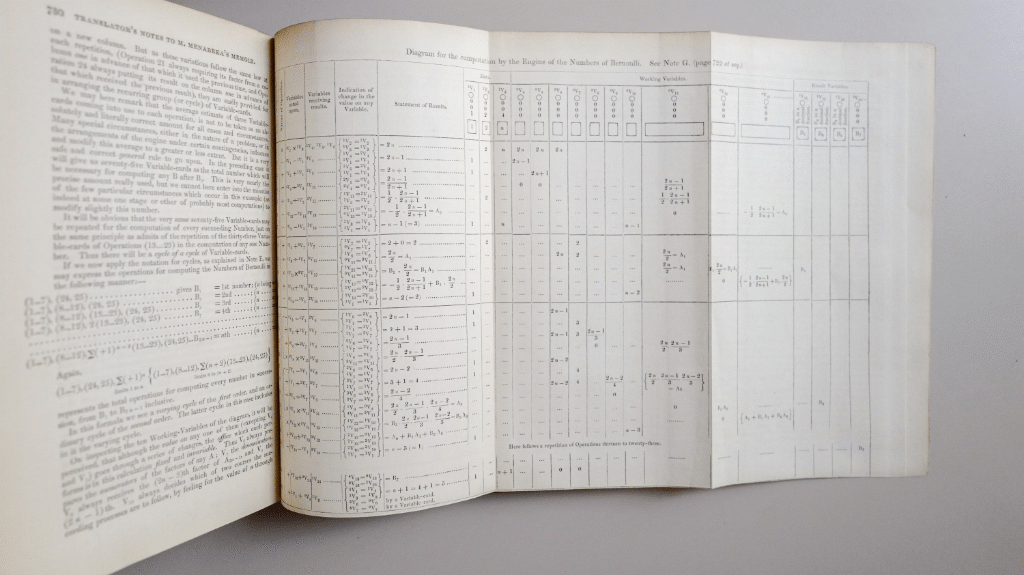Ada Lovelace’s ‘Notes’ contained the first published computer program. More of a trace than an actual listing, it used an iterative algorithm to calculate Bernoulli numbers.Here is the recreated algorithm in Julia. Ada’s original didn’t describe conditions for the repetition of operations 13 to 23, and it wasn’t clear how the array of result variables would actually be populated on the Analytical Engine. However, everything else is pretty much how her program was originally described (apart from fixing Ada’s bug in operation 24).

The results, converting to current notation (Ada’s program calculates B2n), are:

B2 = 1/6
B4 = -1/30
B6 = 1/42
B8 = -1/30
B10 = 5/66
B12 = -691/2730
B14 = 7/6
B16 = -3617/510
B18 = 43867/798
B20 = -174611/330

## An 8-bit Manchester SSEM Simulator

I sometimes give seminars on the history of computing machines and like to use an Elm simulation of an 8-bit version of the Manchester Small-Scale Experimental Machine (SSEM). The one below is useable and there is a full page version here.

It has a memory comprising of 32 8-bit words, an 8-bit accumulator and a 5-bit program counter. Unlike the original it doesn’t increment the program counter before executing the instruction so is a bit easier to describe and program.

The instruction set and encoding is the same as the original machine.

Inst. Description Encoding